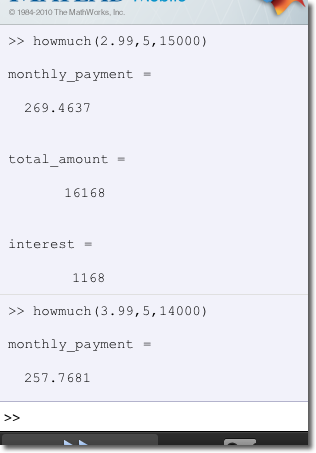# Using MATLAB Mobile to Save Money

This weekend my MATLAB Mobile app more than paid for itself--which is not hard, since it's free! Over the weekend I went shopping for a new car. When it was time to discuss financing, I was presented with a range of rates and promotions. In order to figure out what each combination of rates, loan amount, and length of the loan would cost me, I wrote a simple MATLAB function to do the calculations. Because I have the Financial Toolbox, I took advantage of the payper function, which does all the exponential interest math for me. If you don't have access to that toolbox, it's some basic linear algebra to replicate that calculation.

function howmuch(rate, yrs, amt)
monthly_payment = payper((rate/100)/12,yrs*12,amt)
total_amount = round(monthly_payment*(yrs*12))
interest = total_amount - amt

With this function on my desktop MATLAB's path and the Connector running, I was able to go to the dealership confident that I could make sound financial decisions. For example, one option I had was a 2.99% APR loan, or a 3.99% loan with $1000 cash back. Using my howmuch function, I was able to see that while the second loan has more interest, it was overall cheaper ($15,466 vs \$16,168). An interesting thing I could have also calculated was how long the loan would have to last for the first one to be cheaper.Because I knew that I was going to use this function from MATLAB Mobile, I coded it differently than I normally do. For example, the function does not have semicolons after each command so the values are displayed in the command window. I also used long, descriptive names for those variables since they will be printed too. Normally I would use return values, but that means extra tapping to assign outputs from the mobile command line. For the same reason, I used a function instead of a script, so I would not have to send multiple commands to change the parameter values.

|